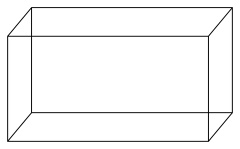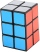# Prism X

The prism with the edges of the lengths x cm, 2x cm, and 3x cm has volume 20250 cm3. What is the area of the surface of the prism?

S =  4950 cm2

### Step-by-step explanation:Did you find an error or inaccuracy? Feel free to write us. Thank you!Savage Math Hacker 21
i think that all math is great but cubes graphic is better.????????Dr Math
graphics fixedTips to related online calculators
Do you want to convert length units?
Tip: Our volume units converter will help you with the conversion of volume units.

#### You need to know the following knowledge to solve this word math problem:

We encourage you to watch this tutorial video on this math problem:

## Related math problems and questions:

• Cuboid edges in ratioCuboid edges lengths are in ratio 2:4:6. Calculate their lengths if you know that the cuboid volume is 24576 cm3.
• BallsThree metal balls with volumes V1=71 cm3 V2=78 cm3 and V3=64 cm3 melted into one ball. Determine it's surface area.
• Cube 1-2-3Calculate the volume and surface area of the cube ABCDEFGH if: a) /AB/ = 4 cm b) perimeter of wall ABCD is 22 cm c) the sum of the lengths of all edges of the cube is 30 cm.
• Minimum surfaceFind the length, breadth, and height of the cuboid-shaped box with a minimum surface area, into which 50 cuboid-shaped blocks, each with length, breadth, and height equal to 4 cm, 3 cm, and 2 cm respectively, can be packed.
• Surface of cubesPeter molded a cuboid 2 cm, 4cm, 9cm of plasticine. Then the plasticine split into two parts in a ratio 1:8. From each piece made a cube. In what ratio are the surfaces of these cubes?
• Regular square prismThe volume of a regular square prism is 192 cm3. The size of its base edge and the body height is 1: 3. Calculate the surface of the prism.
• Cuboid and ratioFind the dimensions of a cuboid having a volume of 810 cm3 if the lengths of its edges coming from the same vertex are in ratio 2: 3: 5
• Prism basesVolume perpendicular quadrilateral prism is 360 cm3. The edges of the base and height of the prism are in the ratio 5:4:2. Determine the area of the base and walls of the prism.
• Two boxes-cubesTwo boxes cube with edges a=38 cm and b = 81 cm is to be replaced by one cube-shaped box (same overall volume). How long will be its edge?
• Cube 6Volume of the cube is 216 cm3, calculate its surface area.
• Three cubesTwo cube-shaped boxes with edges a = 70 cm; b = 90 cm must be replaced by one cube-shaped box. What will be its edge?
• Cuboid edgesThe lengths of the cuboid edges are in the ratio 2: 3: 4. Find their length if you know that the surface of the cuboid is 468 m2.
• Ratio-cuboidThe lengths of the edges of the cuboid are in the ratio 2: 3: 6. Its body diagonal is 14 cm long. Calculate the volume and surface area of the cuboid.
• Volume and areaWhat is the volume of a cube which has an area of 361 cm2?
• PrismRight-angled prism, whose base is a right triangle with leg a = 3 cm and hypotenuse c = 13 cm, has the same volume as a cube with an edge length of 3 dm. a) Find the height of the prism b) Calculate the surface of the prism c) What percentage of the cube'
• Equilateral cylinderEquilateral cylinder (height = base diameter; h = 2r) has a volume of V = 199 cm3 . Calculate the surface area of the cylinder.
• Triangular prismThe triangular prism has a base in the shape of a right triangle, the legs of which is 9 cm and 40 cm long. The height of the prism is 20 cm. What is its volume cm3? And the surface cm2?